# Pair Trading and VAR analysis applied to Energy Stocks

## Pair Trading and VAR analysis applied to Energy Stocks

Updated: Oct 18, 2019This post is a summary of the research paper project created for CQF Institute. If you prefer to read the more detailed original research paper, please click here

Pairs Trading (aka Statistical Arbitrage) was first developed at Morgan Stanley during the 80s and it is based on finding relative mispriced relationships between a pair or group of stocks in
order to benefit from an ultimate convergence towards its theoretical equilibrium. The strategy has several versions relying on multiple methods with the three key branches being the distance method (DM), the cointegration method (CM), and the stochastic spread method (SP). On the one hand, DM is the simplest pair trading strategy with the largest body of research, and consists in calculating the spread between the normalized prices of those possible combinations of stock pairs that have the least sum of squared spreads. Although authors such as Gatev, Goetzmann and Rouwenhorst (2006) found DM to generate positive P&L for the period 1962-2002; Do and Faﬀ (2010) pointed out a that the number of loss-making trades was high and increasing after the turn of the century.

On the other hand, CM is a parametric model based in finding a pair of stocks whose linear combination is stationary i.e. both stocks are said to be cointegrated. Cointegrated pairs are therefore traded based on the stationarity of their spread and short term deviations from the long term equilibrium. Rad, Kwong Yew Low and Faff (2016) conducted a thorough analysis using US stocks’ data from July 1962 to December 2014 comparing DM, CM and copula methods. Their findings highlighted DM delivering a slightly higher monthly return than CM, but CM exhibited a slightly superior Sharpe ratio. Lastly, stochastic spread methods (SP), such as Ohrnstein-Uhlenbeck process, verify the existence of a well-known stochastic model for mean reversion.

Regardless of the method used, the same questions need to be answered to implement an efficient and profitable pairs trading strategy as stated by Vidyamurthy (2004): How do we calculate the spread? How do we identify stock pairs for which such a strategy would work? When can we say that the spread has substantially diverged from the mean?

## EDA (Exploratory Data Analysis)

A sample of 3 stocks and 2 ETFs has been chosen for the period 2000-2018 using daily data from yahoofinance via Python's pandas_datareader library: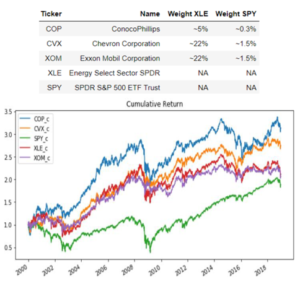The three stocks belong to the Oil & Gas Integrated industry, part of the Energy Sector (MSCI  GSCI Sector Classification). The frequency of the data is daily with observations ranging from 31st December 1999 to 31st December 2018. The three stocks chosen are the top three oil integrated majors by market capitalization and the largest companies in the US Energy sector. In other words, these stocks have identical nature of their operations (integrated oil), similar market capitalization classification (mega large caps), look alike business fundamentals as well as high liquidity in terms of their stock trading turnover that make them ideal candidates for a pair trading. In addition, two ETFs have been added to our sample. On the one hand, Energy Select Sector SPDR seeks to provide an effective representation of the energy sector of the S&P 500 Index. On
the other hand, SPDR S&P 500 ETF Trust allows investors to track the S&P 500 stock market index and is the largest ETF in the world.

Security returns are calculated from the original level prices and multiple statistical studies and density plots are applied to the original returns and a standardized version as displayed below. When comparing all five security returns against a simulated standard normal distribution, the results against the hypothesis of normality are staggering with all securities experiencing abnormal figures of both skewness and kurtosis compared to the automatically generated normal distribution.

## Matrix form estimation for multivariate regression

This section will apply a VAR (Vector Auto Regression) model to security price daily returns. VAR(p) models assume that the passed time series are stationary. Hence, should we want to conduct a direct analysis of non-stationary time series, a standard stable VAR(p) model will not
appropriate. One solution for non-stationary or trending data is to transform it into stationary by first-differencing or some other method

Confirming Stationarity Input

In this way, ADF (Augmented Dickey Fuller) test for stationarity in the security returns confirms that the security returns to be utilized in our VAR(p) model are stationary. The tables displayed below underscore significantly small p-values rejecting the null hypothesis that a unit root is
present aka non-stationarity. In addition, security price differences are also tested for unit root existence and the results also are confirming stationarity of the data. Finally, security price level data is tested as well and, as expected, the results show significant p-values that force us to do not reject the null hypothesis of existence of unit root and, as a result, our VAR(p) analysis will be based on daily price returns as it was intended at the outset.

ADF test results were yielded from a function that uses StatsModels ‘s Python library function adfuller() whereby different ADF lag-driven models are tested using AIC minimization and introducing Schwert (1989) rule
of thumb: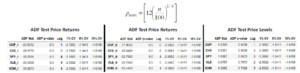Finding Optimal p lag for VAR(p) model

The matrix form for the VAR model can be expresses as it follows for an observation t: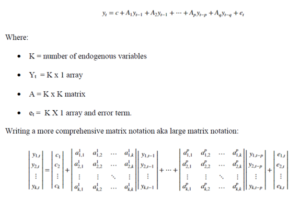After confirming stationarity of the returns calculated for our securities, it is necessary to step forward to the next step i.e. calculate the VAR model optimal number of lags (p). The methodology is straightforward consisting on testing various lags for p and select the value that minimizes a function called the “Information Criterion” (IC).

Optimal lag selection using a single IC might be dangerous as the optimal lag for both AIC and FPE (p=15) yields a very complex model that can be prone to overfitting or only suitable for a single specific purpose (describe or forecast). When considering BIC and HQIC's optimal lags the models are much less complex. As a result, an IC score ranking is calculated (the higher the IC, the higher the score) to gather different insights from the multiple IC considered and the results’ ranking displayed in the next table were favoring the use of a VAR model with 2 lags: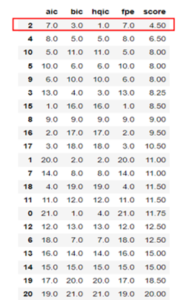Next step is to run the VAR model using the optimal 2 lags. For this purpose, a coded proprietary function has been used using matrix formulation and the results are compared against the results of a VAR (2) run using Python’s well-known StatsModels library. The coefficients obtained from both the proprietary coded VAR(2) function and SM function are highlighted in the next table with identical results: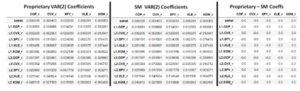Regarding coefficients’ stability is tested by requiring eigenvalues of each relationship matrix A to be inside the unit circle (<1). Using the VAR (2) model via SM library the results are positive confirming the condition above of coefficient stability in our VAR(2) model.

## Forecasting capability of regression with IRF and Granger Causality

IRF (Impulse Response Function)

Impulse responses are the estimated responses to a unit impulse in one of the variables. Our VAR(2) exhibited significantly high correlation between residuals. In other words, IRF assumption that shocks occur isolatedly i.e. only one variable experiences a shock on a period - is not realistic according to our results. In fact, financial markets are featured by tail-risk events during which variables tend to break historical correlation rules of thumb and misbehave increasing their correlations punctually. In this scenarios IRF analysis using MA expression is very useful as an orthogonalized transformation is carried out to obtain IRF coefficients that contain the aforementioned correlation effect. VAR model therefore is transformed into the next expression: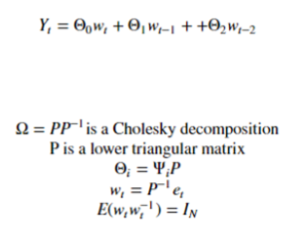The results for orthogonalized IRF are shown below and point out a significant fade away effect after the initial impulse across the board for both marginal and cumulative shocks for a period of 5 days: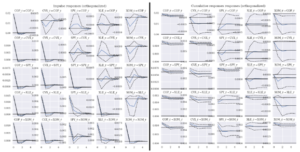Granger Causality Analysis
Granger causality test was firstly proposed in 1969 and is a statistical test for studying causality between two different variables. The null hypothesis is that the coefficients corresponding to past values of the “causing” variable are zero i.e. the null hypothesis for the equation below - p and q being the shortest and longest significant lag lengths for x - will be: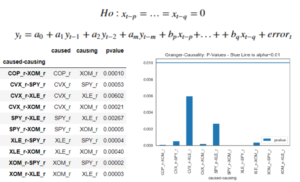Granger causality test works under the assumption that all the VAR variables are stationary (confirmed earlier in this post). Granger Causality tests are conducted for all the pair combinations possible for all our five securities with only the pairs above surviving for a significance level of 10% i.e. only pair combinations where p-value is below 10% are shortlisted or, in other words, only those were the null hypothesis of non-Granger Causality is rejected are considered.

## Engle-Granger procedure and cointegrated pair identification.

Two or more series are cointegrated if a linear combination exists which makes the residuals integrated of order zero I(0). Engle–Granger (EG) will be used here to test a bivariate cointegration relationship. This method is based on testing that the residuals from the regression model between the securities y and x shown below are stationary: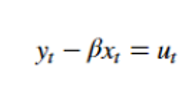The training sample to be considered will be the first two thirds of data from the total period 2000-2018 i.e. sample ranging from 31st December 1999 to 30th April 2013. Moreover, a 5% significance level is used to filter p-values obtained from ADF tests in order to shortlist relevant pair cointegration combinations.

As mentioned before, EG will be used in this project in order to ascertain whether or not two securities in our sample are cointegrated:

Step 1: Obtain fitted residuals to conduct ADF test (Stationarity Test)

The combinations between XLE, XOM and COP price levels exhibit stationarity passing the ADF acid test with no unit root in their residuals assuming 1% significance level. The other pairs involving SPY and CVX need first differences to pass Engle Granger test with the exception of COP-SPY. XOM-COP, XLE-XOM and XOM-XLE are particularly relevant as these pair combinations were also relevant in our preliminary IRF and Granger Causality Analysis.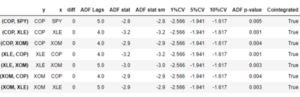Step 2: ECM using residuals from Step 1

After confirming the pairs and degree of differences where we found cointegrated relationships i.e. residuals were stationary, now it's time to use the residuals to obtain the ECM (Equilibrium Correction Form). For this purpose, the pair COP-XOM has been selected due to its high statistical significance as aforementioned. ECM (Equilibrium Correction Model) is obtained as: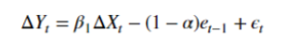Where et-1 are the residuals from the static equilibrium model lagged one period. This second step is helpful to understand if there's an equilibrium correction model (ECM) effectively working i.e. the parameter –(1-α) is interpreted as the speed of correction towards the equilibrium level. In fact, this parameter significance will also aid to select which pair order is more relevant. For instance, for the combination of COP and XOM the next two ECM regressions are provided to check whether COP-XOM or XOM-COP is the most relevant pair.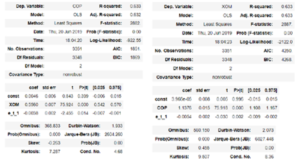Testing the null hypothesis: −(1−α)=0, the lagged residual coefficient p-value is not significant for significance levels below 2% for both pairs. In other words, we can assume that there's a correction mechanism helping the spread to reach long term equilibrium with a level of confidence of 97%. However, XOM-COP pair equilibrium correction mechanism seems to be more reliable than COP-XOM with a p-value even below 1%.

## Strategy Backtesting

A proprietary-coded Python class and some add-on helper functions were used for backtesting and performance analysis purposes of several pair trading strategies for the selected pair XOM-COP. In this way, Engle-Granger two-step procedure is necessary to ensure cointegration of the pair series along with stationarity of the spread: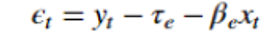In order to find out the optimal entry and exit points, this spread or residual of the cointegration relationship needs to be modelled. In this project a Ornstien-Uhlenbeck (OU) process is used to model the spread and identify entry/ exit points. In this way, after checking for stationarity, the spread OU process has the next solution for its SDE (stochastic differential equation) as displayed below: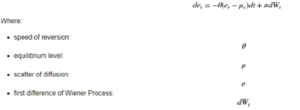The parameters expressions obtained are used along with all our data to build a backtesting using 3 periods: in-sample period, out-of-sample period and total period. The most useful periods to watch are the out-of-sample as it generalizes the findings from in-sample period parameter optimization as well the “total period” backtesting as it provides a lengthy time span to test the effectiveness of the strategy.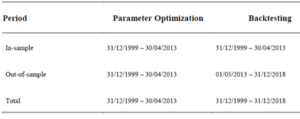The backtesting trading rule rationale is summarized in the next lines using pseudo code format. Bottom line, the strategy is mean-reverting using the pair spread compared to our entry/exit points - obtained from in-sample analysis – to go long or short and, eventually, close the position back to neutral when a reversion to the mean occurs: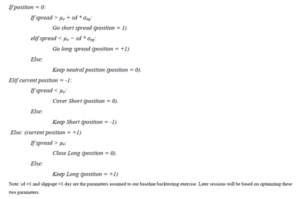Backtesting Results
Several default assumptions are introduced in order to set forth a more realistic framework to interpret the results:
• The multiplier sd applied to σeq to obtain entry points is assumed to be equal to 1.
• The time between trading signal and trade execution is 1 day aka slippage period.
• Risk free rate assumption for Sharpe ratio calculations is 2%.
• Transaction fee rate is set up to 17 bps in line with other body of research.
The results from applying the strategy to these three different backtesting periods are shown in the next tables and charts: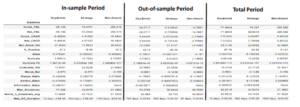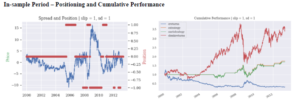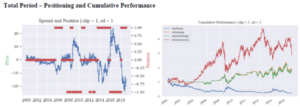Optimization

Moreover, an optimization exercise is applied to obtain both the spread’s multiple applied to σeq (sd in the pseudo code presented earlier) and slippage parameters that maximizes the strategy performance. The optimization splits the total period from 2000 to 2018 in three equal subperiods and calculates key metrics multiple combinations of sd (1 to 5 with 0.5x steps) and slippage (1 to 3 days considered) returning the best parameter combination for the strategy on each subperiod.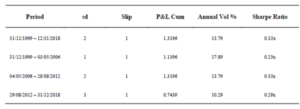Risk Factors Exposure
This section presents a risk factor performance attribution analysis using Fama-French 2014 5-factor model comprehending Market (Market-Risk Premium), SMB (Size Premium), HML (Value Premium), RMW (Operating Profitability Premium) and CMA (Low Capital Expenditure Intensity Premium). Moreover, Fama-French Momentum Factor has been also added to the model as several practitioners and scholars have criticized the no inclusion of the long-time accepted momentum effect in their overall 5-Factor model. Lastly, two additional factors are included to represent macro risk (USD Risk Premium) and industry/sector premium (Energy Sector Risk Premium).

Important to point out that only periods with either long or short positions in the XOM-COP spread are considered, ignoring periods where the strategy is in neutral mode. In the same fashion as it was done in past sections, we conducted risk factor analysis for three periods: total period (2000-2018) as well as our in-sample (2000-2013) and out-of-sample (2013-2018).

Several remarks can be extracted from the analysis in the charts below: Firstly, only a group of risk premium are persistent throughout time witnessing their p-values below 10% significance level: momentum (MOM) and Sector risk premium (LOC). From these couple of factors, only momentum’s factor sign is unchangeable in negative territory across all the periods. In other words, XOM-COP trading strategy is negatively exposed to periods where outperformance in momentum style prevails.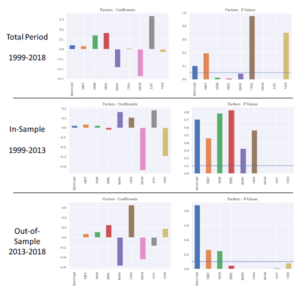Other significant risk exposures arise when paying attention to the total period returns from 1999 to 2018. Apparently, the strategy has a positive exposure to traditional size (SMB) and value (HML) risk premiums whereas it’s negatively exposed to quality factors such as Operating Profitability Premium (RMW). That said, these exposures are less persistent in time that the previously highlighted negative sensitivity to momentum (MOM).